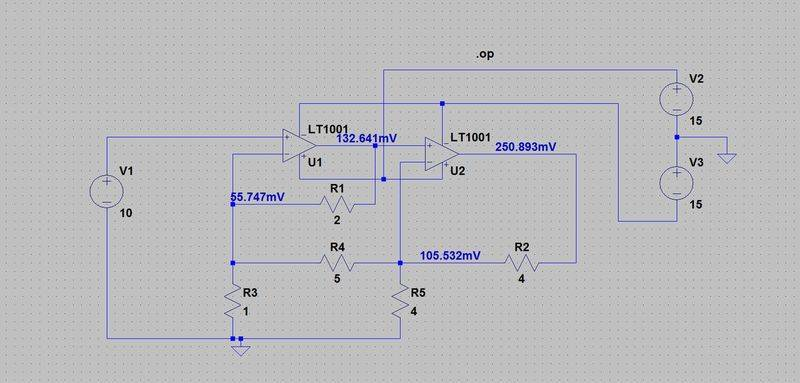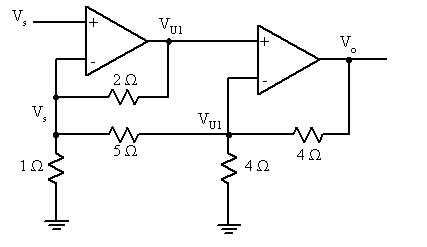# Opamp Circuit Analysis

• Engineering

## Homework Statement

Find the voltage gain, V0/Vs, for the circuit below. All resistors are in Ohms.## Homework Equations

I identified this as a non inverting amplifier. Therefore:
$$V_{0}=\frac{R_{S}+ R_{f}}{R_{s}}V_g$$

## The Attempt at a Solution

Vo = 8/3*V1 (v1 = output of U1)
V1 = 29/900*vs

V0 = 58/675*Vs

This does not agree with LT Spice. I am not sure how to break this apart to analyze it.

gneill
Mentor
On your simulation, back off on the input voltage significantly: Try something like 0.01 V. That's 10 mV.
At 10V input, I think that the LT1001 is going to go into short-circuit protection mode if you try to force the pair of them to produce something on the order of 10 amps of output current.

In order to obtain the amplification analytically, try nodal analysis.

•1 person
AlephZero
Homework Helper
It the LTSpice simulation is using a "real" model of the op amp, your resistor values are much too small to be practical. Try increasing all the resistors by a factor of 10,000 and see if the results look more sensible.

You might need to reduce the input voltage as well. Try say 1V with the higher resistors.

If you just reduce the input to 0.01V and leave the resistors small, the LTSpice results will probably be affected by the differences between the real op amp model and an ideal op amp, so they won't agree with your hand calculations.

I think that the LT1001 is going to go into short-circuit protection mode if you try to force the pair of them to produce something on the order of 10 amps of output current.

A quick google search for the LT1001 data sheet suggests it is meant to work with currents less than 10 mA, not 10A.

Any tips on how to deal with that 5 ohm resister between the two?

gneill
Mentor
Any tips on how to deal with that 5 ohm resister between the two?

Nodal analysis will take care of it very nicely.

Vs = V(R3) and V(U1) = V(R5)

For the left node
$$-V_{s}+ \frac{V_{U1}-V_{s}}{2}+ \frac{V_{U1}-V_{s}}{5}=0$$

And the right node

$$-\frac{V_{0}}{4}+ \frac{V_{U1}}{4} + \frac{V_{U1}-V_{s}}{5}=0$$

So for V(U1) = (17/7) Vs, which agrees with the simulation.

I have went through the second part for V0 and get V0 = 25/17 V(U1) = 25/7 Vs in both case are incorrect.

If I add 1 to the 25/17 then with the 17/7 for V(U1) i get a overall gain of 6 which works but not seeing where i missed it.

gneill
Mentor
Can you explain a bit about your second node equation? Your first node equation seems to be adding the currents entering the node, while the second seems to be adding currents leaving the node. This is fine, but I'm not seeing where the first term, -Vo/4 is coming from.

Here's your circuit with the power supply lines removed (to make it a bit easier to see the relevant component connections) and what I think are your voltage designations for the relevant nodes:#### Attachments

•1 person
That looks like the problem. Should be (V0 - V(U1) )/4 entering the node. then V(U1)/4 and (V(U1) - Vs)/5 leaving the node.